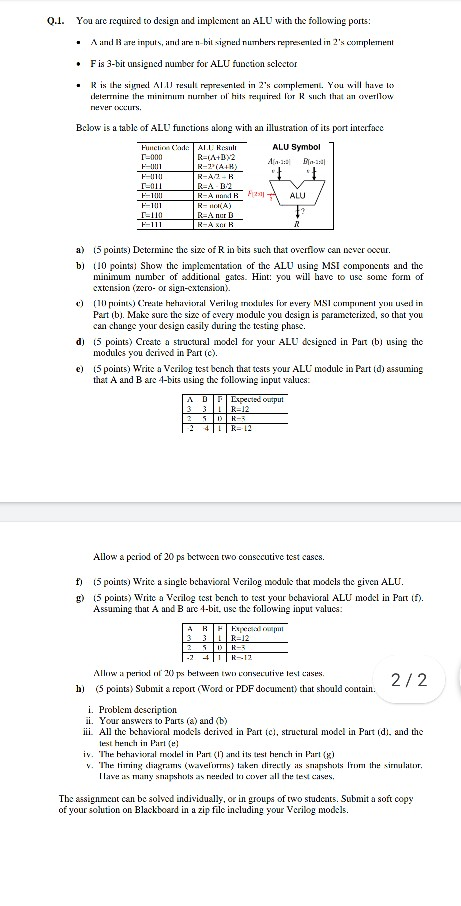# (Solved) : Required Design Implement Alu Following Ports B Inputs N Bit Signed Numbers Represented 2 Q42767745 . . .

You are required to design and implement an ALU withthe following ports:
• A and B are inputs, and are n-bit signed numbers represented in2’s complement
• F is 3-bit unsigned number for ALU function selector
• R is the signed ALU result represented in 2’s complement. Youwill have to
determine the minimum number of bits required for R such that anoverflow
never occurs.
Below is a table of ALU functions along with an illustration of itsport interface
Function Code ALU Result
F=000 R=(A+B)/2
F=001 R=2*(A+B)
F=010 R=A/2 + B
F=011 R=A – B/2
F=100 R=A nand B
F=101 R= not(A)
F=110 R=A nor B
F=111 R=A xor B
a) (5 points) Determine the size of R in bits such that overflowcan never occur.
b) (10 points) Show the implementation of the ALU using MSIcomponents and the
minimum number of additional gates. Hint: you will have to use someform of
extension (zero- or sign-extension).
c) (10 points) Create behavioral Verilog modules for every MSIcomponent you used in
Part (b). Make sure the size of every module you design isparameterized, so that you
can change your design easily during the testing phase.
d) (5 points) Create a structural model for your ALU designed inPart (b) using the
modules you derived in Part (c).
e) (5 points) Write a Verilog test bench that tests your ALU modulein Part (d) assuming
that A and B are 4-bits using the following input values:
A B F Expected output
3 3 1 R=12
2 5 0 R=3
-2 -4 1 R=-12
Allow a period of 20 ps between two consecutive test cases.
f) (5 points) Write a single behavioral Verilog module that modelsthe given ALU.
g) (5 points) Write a Verilog test bench to test your behavioralALU model in Part (f).
Assuming that A and B are 4-bit, use the following inputvalues:
A B F Expected output
3 3 1 R=12
2 5 0 R=3
-2 -4 1 R=-12
Allow a period of 20 ps between two consecutive test cases.
h) (5 points) Submit a report (Word or PDF document) that shouldcontain:
i. Problem description
iii. All the behavioral models derived in Part (c), structuralmodel in Part (d), and the
test bench in Part (e)
iv. The behavioral model in Part (f) and its test bench in Part(g)
v. The timing diagrams (waveforms) taken directly as snapshots fromthe simulator.
Have as many snapshots as needed to cover all the test cases.Answer to You are required to design and implement an ALU with the following ports: • A and B are inputs, and are n-bit signed n…

We are the best freelance writing portal. Looking for online writing, editing or proofreading jobs? We have plenty of writing assignments to handle.